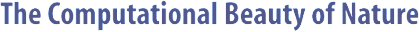Computer Explorations of Fractals, Chaos,Complex Systems, and Adaptation· title page · home* · cover artwork · jacket text · table of contents · the author* · ordering informationBook Contents
 · three themes · part synopses · selected excerpts · all figures from book · quotes from book · glossary from book · bibliography · slide showSource Code
 · overview &documentation · FAQ list* · download source code · java appletsMiscellany
 · news* · reviews & awards · errata · for educators · bibliography (BibTeX format) · other linksROSSLER Documentation

```

```

#### NAME

```       rossler - plot the phase space of the Rossler system

```

#### SYNOPSIS

```       rossler -help
or
rossler
[-width  integer] [-height integer] [-skip integer]
[-points integer] [-A double] [-B double] [-C  dou-
ble]  [-delta  integer]  [-dt  double] [-x0 double]
[-y0 double] [-z0 double] [-data] [-xp string] [-yp
string]  [-factor  double]  [-inv]  [-mag  integer]
[-term string]

```

#### DESCRIPTION

```       The phase space of the Rossler system, which is  described
by the three differential equations

dx/dt = -y - z,

dy/dt = A * y + x, and

dz/dt = B + x * z - C * z,

is  plotted  according to the specified parameters.  Valid
arguments passed with the -xp and -yp options can  be  any
one  of  x(t), y(t), z(t), x(t-delta), y(t-delta), or z(t-
delta).  Thus, the displayed plot can take the form  of  a
state space plot or a delayed coordinate plot.

```

#### OPTIONS

```       -width integer
Width of the plot in pixels.

-height integer
Height of the plot in pixels.

-skip integer
Number of initial points to skip.

-points integer
Number of points to plot.

-A double
Value of the A parameter.

-B double
Value of the B parameter.

-C double
Value of The C parameter.

-delta integer
Time delay term.

-dt double
Time step.

-x0 double
Initial X value.

-y0 double
Initial Y value.

-z0 double
Initial Z value.

-data  Don't plot, but print points.

-xp string
X-coordinate for plot.

-yp string
Y-coordinate for plot.

-factor double
Auto-scale expansion factor.

-inv   Invert all colors?

-mag integer
Magnification factor.

-term string
How to plot points.

```

#### MISCELLANY

```       The  plot region is determined by the points that are ini-
tially skipped.  If this number is too small (i.e., it  is
not  very  representative of the range of the plotted val-
ues), then you may need to increase the  number  specified
by  the  -skip  option.  Alternatively, you can adjust the
value  given  to  -factor,   which   simply   fractionally
increases the border of the plot.

The  program uses a second-order Euler's method to perform
the numerical integration, which is sufficient for  simple

```

#### BUGS

```       No  sanity  checks  are performed to make sure that any of
the options make sense.

```

#### AUTHOR

```       Copyright (c) 1997, Gary William Flake.

Permission granted for any use according to  the  standard
GNU ``copyleft'' agreement provided that the author's com-
ments are neither modified nor removed.   No  warranty  is

given or implied.

```Copyright © Gary William Flake, 1998-2002. All Rights Reserved.Last modified: 30 Nov 2002